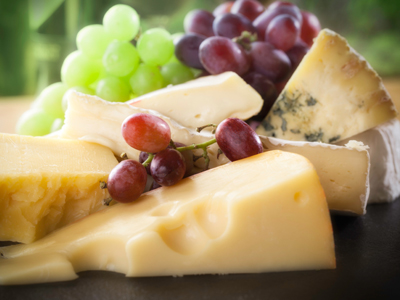Joshua buys half a kilo of cheese and Oliver buys 250 grams of cheese.

# Level 3-4 Shapes - Measuring Mass

As part of your work in KS3 Maths on shapes, you will be asked to do some measurements. You'll be used to measuring areas, distances (like width or length) and volumes or capacities. One other thing you will have to measure is weight or mass.

Technically, there is a difference between 'weight' and 'mass', as you'll learn in your Physics classes. But in real life the two terms are usually taken to mean the same thing. As far as maths is concerned, both are measured in the same units - grams (g), kilograms (kg) and tonnes. There are 1,000 grams in a kilogram and 1,000 kilograms in a tonne so converting between them is quite simple - just divide or multiply by 1,000. You may also come across imperial units of weight, like ounces, pounds or tons. Don't worry - this quiz deals only with metric weights. There's a quiz all about imperial weights which you're no doubt looking forward to playing! Why not try it after this?

See how much you know about measuring mass metrically in the following quiz.

1.
Ruby has 3 kilos of sugar, Megan has half as much as Ruby and Ellie has half as much as Megan. How much sugar does Ellie have?
1.5 k
1.0 k
750 g
500 g
Don't forget that k = kilo and g = gram
2.
How many grams are there in one kilogram?
10
100
1,000
1,000,000
'Kilo' means 'thousand'
3.
Peter has collected a number of 1p coins and he finds that one coin weighs 313 grams. He then weighs all the coins together and they weigh 666 grams. Approximately how much are the pennies worth?
50p
£1.00
£1.50
£2.00
666g ÷ 31?3 = 199.8 which we can round up to 200. So, 200 coins each worth 1p means that Peter's collection is worth £2.00
4.
How many grams are there in 13 kilograms?
130
1,300
13,000
130,000
Just multiply 13 x 1,000 for the answer
5.
What is the name given to 1,000 kilograms?
tun
ton
tonne
tunne
A 'ton' is the name given to 2,240 pounds in imperial measurement
6.
Charlotte buys two jars of jam each weighing 400 grams, two bags of sugar each weighing a kilo and three tubs of yoghurt each weighing 100 grams. What is the total weight of her shopping?
2.9 kilos
3.1 kilos
3.3 kilos
4.0 kilos
Make sure that you understand that a kilo is 1,000 grams and that 400 grams is 0.4 of a kilo
7.
A lorry is delivering to shops and at the start of the day the lorry contains 10 tonnes of potatoes. The first shop takes 500 kilos of potatoes and the second shop takes 2.5 tonnes of potatoes. How many potatoes are left on the lorry?
5.0 tonnes
7.0 tonnes
7.5 tonnes
9.5 tonnes
500kg = 0.5 tonnes so 10 - 0.5 - 2.5 = 7 tonnes
8.
What is the name given to 100 kilograms?
Megagram
Mellagram
Monogram
There is no name for 100 kilograms
Metric weights go up in thousands, not hundreds
9.
Emily weighs 4.5 kilos more than Sophie. What is the difference in their weights in grams?
450
2,250
4,500
9,000
Multiply 4.5 x 1,000 for the answer
10.
Joshua buys half a kilo of cheese and Oliver buys 250 grams of cheese. What is the difference between these two amounts?
150 g
200 g
250 g
300 g
Half a kilo = 500 grams. The abbreviation g = grams